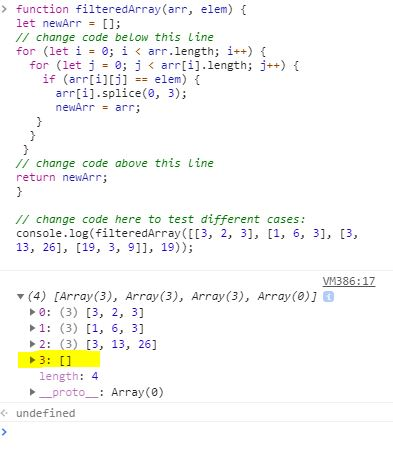# Iterate Through All an Array's Items Using For Loops - Code seems to work. but test won't pass

Setup:

``````function filteredArray(arr, elem) {
let newArr = [];
// change code below this line

// change code above this line
return newArr;
}

// change code here to test different cases:
console.log(filteredArray([[3, 2, 3], [1, 6, 3], [3, 13, 26], [19, 3, 9]], 3));
``````

My objective is to:
“Modify the function, using a `for` loop, to return a filtered version of the passed array such that any array nested within `arr` containing `elem` has been removed.”

My solution:

``````function filteredArray(arr, elem) {
let newArr = [];
// change code below this line
for (let i = 0; i < arr.length; i++) {
for (let j = 0; j < arr[i].length; j++) {
if (arr[i][j] == elem) {
arr[i].splice(0, 3);
newArr = arr;
}
}
}
// change code above this line
return newArr;
}
``````

Problem:
The code seems to do the trick as it removes nested arrays containing elem, but when I press “run the test” it doesn’t pass the test. Can anyone give me a hint as to what the problem might be?

I suggest you try looking at the returned value for all the tests using `console.log(JSON.stringify(...))` so you can see what they are returning

Or you can look at what happens step by step in your code using this tool: http://pythontutor.com/javascript.html

That’s a really cool tool. Thanks for sharingI’ve tried using it, but again…the code seems to work (or I am too inexperienced to realise where it went wrong). The only thing I can see that might be a problem, is the fact that the code doesn’t seem to remove the actual nested array, but only the elements within it. Which might be, why it doesn’t pass the test?Well, there you have an empty array, that one shouldn’t be there. You need to completely remove the array